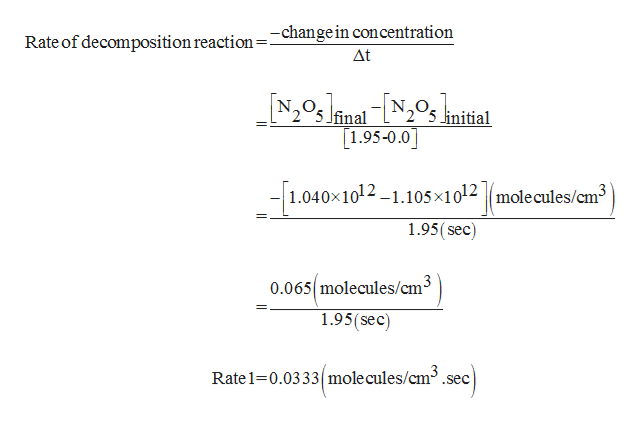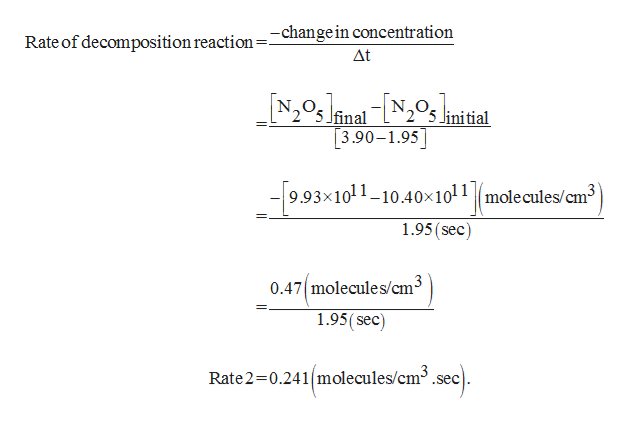Dinitrogen pentoxide (N2O5) decomposes as follows to nitrogen dioxide and nitrogen trioxide:N2​O5​(g)NO2​(g)+NO3​(g)Calculate the average rate of the reaction between consecutive measurement times in the following table. Time (s)[N2O5] (molecules/cm3) 0.001.105×1012  1.95 1.040×1012 3.90 9.93×1011 5.85 9.58×1011 7.80 9.33×1011  Express every answer to two significant figures.Rate 1 =     molecules/(cm3·s)    Rate 2 =    molecules/(cm3·s)     Rate 3 =    molecules/(cm3·s)   Rate 4 =    molecules/(cm3·s)

Question
Dinitrogen pentoxide (N2O5) decomposes as follows to nitrogen dioxide and nitrogen trioxide:

N2​O5​(g)NO2​(g)+NO3​(g)

Calculate the average rate of the reaction between consecutive measurement times in the following table.
 Time (s) [N2O5] (molecules/cm3) 0.00 1.105×1012 1.95 1.040×1012 3.90 9.93×1011 5.85 9.58×1011 7.80 9.33×1011

Express every answer to two significant figures.
Rate 1 =     molecules/(cm3·s)

Rate 2 =    molecules/(cm3·s)

Rate 3 =    molecules/(cm3·s)

Rate 4 =    molecules/(cm3·s)
Step 1

Rate of reaction is calculated using the given formula,

Step 2

Rate 1 is calculated as shown,help_outlineImage TranscriptioncloseRate of decomposition reaction =-changein concentration Δt Ifinal linitial 1.95-0.0 1.040x1012-1.105x1012 |molecules/cm3 1.95(sec) 0.065 molecules/cm3 1.95(sec) Rate 1 0.0333 mole cules/cm3.sec fullscreen
Step 3

Rate 2 is calculate...help_outlineImage TranscriptioncloseRate of decomposition reaction=_changein concentration Δt NinaNiial final ini tial 3.90-1.95 9.93x1011-10.40x1011 molecules/cm3 1.95(sec) 0.47molecules/cm3 1.95(sec) Rate 2 0.241 molecules/cm3.se fullscreen

Want to see the full answer?

See Solution

Want to see this answer and more?

Our solutions are written by experts, many with advanced degrees, and available 24/7

See Solution
Tagged in

Chemistry